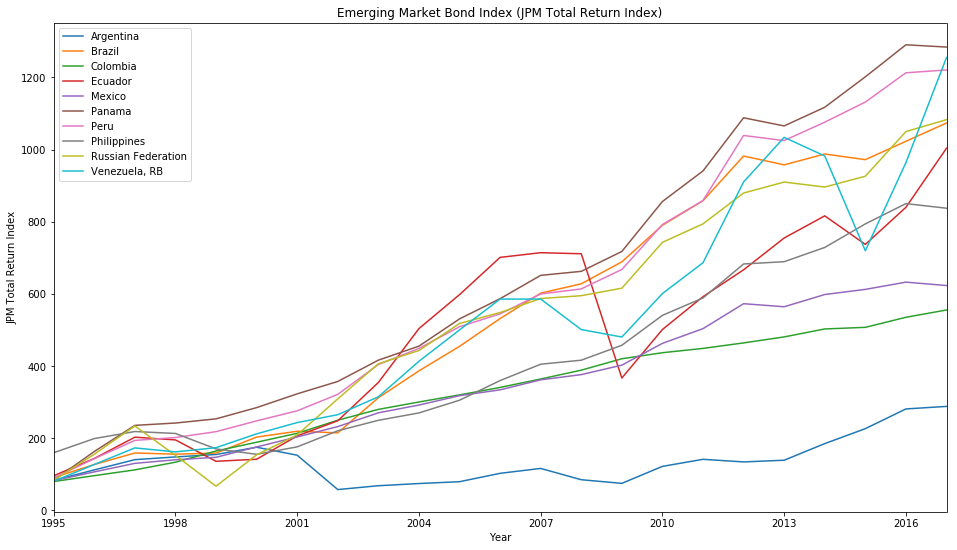Image from Wikimedia Commons

# Working with Missing Values in Pandas

Here we’ll see how to work with missing values in a Pandas DataFrame on a data set from the World Bank Open Data of the Global Economic Monitor.

import pandas as pd

path = 'data/GemDataEXTR/Emerging Market Bond Index (JPM Total Return Index).xlsx'

# Drop first row
df = df.iloc[1:]

# Convert index column to integer
df.index = df.index.map(int)

Argentina Bulgaria Belarus Belize Brazil Chile China Cote d'Ivoire Colombia Developing Countries ... El Salvador Serbia Trinidad and Tobago Tunisia Turkey Ukraine Uruguay Venezuela, RB Vietnam South Africa
1990 NaN NaN NaN NaN NaN NaN NaN NaN NaN NaN ... NaN NaN NaN NaN NaN NaN NaN NaN NaN NaN
1991 NaN NaN NaN NaN NaN NaN NaN NaN NaN NaN ... NaN NaN NaN NaN NaN NaN NaN NaN NaN NaN
1992 NaN NaN NaN NaN NaN NaN NaN NaN NaN NaN ... NaN NaN NaN NaN NaN NaN NaN NaN NaN NaN
1993 NaN NaN NaN NaN NaN NaN NaN NaN NaN NaN ... NaN NaN NaN NaN NaN NaN NaN NaN NaN NaN
1994 NaN NaN NaN NaN NaN NaN NaN NaN NaN NaN ... NaN NaN NaN NaN NaN NaN NaN NaN NaN NaN
1995 82.61642 120.3042 NaN NaN 90.6030 NaN NaN NaN 79.44512 NaN ... NaN NaN NaN NaN NaN NaN NaN 80.24534 NaN NaN
1996 111.72060 156.6395 NaN NaN 126.5848 NaN NaN NaN 95.76585 NaN ... NaN NaN NaN NaN NaN NaN NaN 126.69840 NaN NaN
1997 140.11080 249.7464 NaN NaN 158.6840 NaN NaN NaN 111.81540 NaN ... NaN NaN NaN NaN NaN NaN NaN 172.71890 NaN NaN
1998 147.66550 281.3943 NaN NaN 155.3104 NaN NaN NaN 133.09940 NaN ... NaN NaN NaN NaN NaN NaN NaN 161.67040 NaN NaN
1999 154.43290 305.8159 NaN NaN 158.3479 NaN NaN NaN 164.19570 NaN ... NaN NaN NaN NaN 138.8067 NaN NaN 173.32320 NaN NaN
2000 174.57410 370.4026 NaN NaN 202.6921 NaN NaN NaN 188.40200 NaN ... NaN NaN NaN NaN 152.3002 NaN NaN 211.69040 NaN NaN
2001 152.42480 410.3846 NaN NaN 218.9289 NaN NaN NaN 213.20560 NaN ... NaN NaN NaN NaN 149.6182 180.0858 NaN 242.96080 NaN NaN

12 rows × 49 columns

As you can see the data set is filled with quite a few NaN values. In order for us to work properly with the data set we will drop all rows where all values missing by using the pandas.DataFrame.dropna function.

df.dropna(axis=0, how='all', inplace=True)


We can check with Pandas how many values are still null by summing over result of the pandas.isnull function. For the summing we use pandas.DataFrame.sum function to sum over an axis (by default axis 0) which returns us a pandas.Series. Note that in the sum all True values are viewed as 1 and all False values as 0. Now we can see the first 10 countries with their number of missing values.

df.isnull().sum()[:10]

Argentina                0
Bulgaria                 4
Belarus                 19
Belize                  18
Brazil                   0
Chile                   18
China                   18
Cote d'Ivoire           18
Colombia                 0
Developing Countries    18
dtype: int64


This function is also a great way to get a quick overview in which columns are missing values hidden.

Say, we want to get all the countries that have no missing values. We get those by first getting the Series with the number of each of the missing values from before and then applying a condition with the pandas.Series.where function followerd by dropping all the resulting countries which are NaN. Another way is to filter the Series is by using boolean variables s == 0 which evaluates the whole Series if each value is equals to zero by a boolean value. This boolean Series can be then in turn used to filter the Series by applying s[s == 0] which does the same thing as the previous example.

s = df.isnull().sum()
countries = s.where(s == 0).dropna(how='any') # eqivalent
countries = s[s == 0]                         # eqivalent


# Visualize Data

Finally we will plot part of the data set with the pandas.DataFrame.plot function and we will compare the Emerging Market Bond Index (JPM Total Return Index) for all countries that have no missing values in the data set. We do that by filtering this time the DataFrame by the columns with the country names that we extracted perviously.

%matplotlib inline
import matplotlib

# Specify figure size
matplotlib.rcParams['figure.figsize'] = (16, 9)

ax = df[countries.index].plot(title='Emerging Market Bond Index (JPM Total Return Index)');
ax.set_xlabel('Year');
ax.set_ylabel('JPM Total Return Index');

# Force integer labels for x-axis
ax.xaxis.set_major_locator(matplotlib.ticker.MaxNLocator(integer=True));